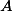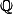# Martin Orr's Blog

## Universal vector extensions of abelian varieties

Posted by Martin Orr on Friday, 09 May 2014 at 18:25

In the last post we showed that extensions of an abelian varietyby the additive groupare classified by a vector spacewhich is canonically isomorphic to. In this post I will show that there is a so-called universal vector extension of, that is, a vector extensionofsuch that every vector extension ofcan be obtained in a unique way as a pushout of. The vector group part ofis, and an ingredient which we require from last time is that this is finite-dimensional.

Even if you are not interested in vector extensions of abelian varieties for their own sake (and I am not), they still have interesting properties. For example, I will show that they provide examples of algebraic varieties over the complex numbers which are non-isomorphic as algebraic varieties but which are isomorphic as complex manifolds.

## Vector extensions of abelian varieties

Posted by Martin Orr on Thursday, 17 April 2014 at 18:12

A theme of my posts on abelian varieties has been ad hoc constructions of objects which are equivalent to the (co)homology of abelian varieties together with their appropriate extra structures -- the period lattice for singular homology and the Hodge structure, the Tate module for-adic cohomology and its Galois representation. I want to do the same thing for de Rham cohomology. To prepare for this, I need to discuss vector extensions of abelian varieties -- that is extensions of abelian varieties by vector groups.

In this post I will define and classify extensions of an abelian variety by the additive group. We will conclude that, the set of isomorphism classes of such extensions, forms a vector space isomorphic to the tangent space of the dual of. Most of this was discovered by Rosenlicht in the 1950s.

## Endomorphisms of simple abelian varieties

Posted by Martin Orr on Thursday, 03 January 2013 at 15:51

Today I will discuss the classification of endomorphism algebras of simple abelian varieties. The endomorphism algebra of a non-simple abelian variety can easily be computed from the endomorphism algebras of its simple factors. For a simple abelian variety, its endomorphism algebra is a division algebra of finite dimension over. (A division algebra is a not-necessarily-commutative algebra in which every non-zero element is invertible.) As discussed last time, the endomorphism algebra also has a positive involution, the Rosati involution. There may be many Rosati involutions, coming from different polarisations of the abelian variety, but all we care about today is the existence of a positive involution. Division algebras with positive involutions were classified by Albert in the 1930s.

## Rosati involutions

Posted by Martin Orr on Wednesday, 05 December 2012 at 16:04

I intend to return to the basic theory of abelian varieties and write write a few posts on their endomorphism algebras and associated moduli spaces. To begin with, I will discuss the Rosati involution which is an involution of the endomorphism algebra coming from a polarisation. The existence of such an involution is crucial for the classification of endomorphism algebras which I will discuss next.

## Dijkstra on the pigeonhole principle

Posted by Martin Orr on Thursday, 04 October 2012 at 17:41

The computing scientist Edsger Dijkstra did not like the standard formulation of the pigeonhole principle:

Ifcompartments containobjects, then at least one compartment contains at least two objects.

He preferred:

For a non-empty, finite bag of numbers, the maximum value is at least the average value.

Of course there are times when Dijkstra's principle is preferable to the traditional statement above (and to its generalisation which I will state below) but I think that there are also times when the traditional statement is preferable, and I am going to counter some of Dijkstra's arguments below. These come from EWD980 and EWD1094.

I am thinking about this today because I am going to teach the pigeonhole principle to some sixth formers at the Maths Olympiad Club at Orsay on Saturday, and I have already taught it twice at the National Mathematics Summer School in Birmingham.

Tags maths, nmss, teaching Read more...